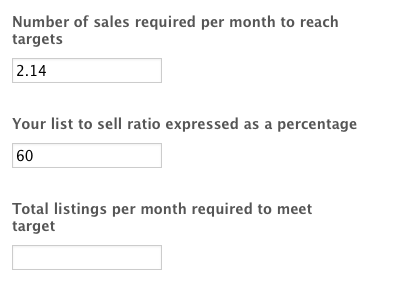# Formula for Calculator

•a1119989
Asked on May 20, 2014 at 02:26 AM

Hi there,

On this form:  http://www.jotformpro.com/form/41380807749967

I need the last field to calculate the following problem:

Number of sales required per month to reach target = 2.14 (see example attached)

List to sell ratio = 60% expressed as a decimal 0.6 (this means the agent sells 60% of the houses they list)

So for the last field I need it to calculate the following formula:

If "Number of sales required per month to reach target" = 60%  expressed as a decimal 0.6(list to sell ratio) then what is 100% of "Number of sales required per month to reach target" (to be calculated in the Total listings per month required to meet target field)

Thanks for your help

Page URL:
http://www.jotformpro.com/form/41380807749967•Ashwin
Answered on May 20, 2014 at 07:38 AM

Hello a1119989,

It seems you have created several threads for the same question. I have already responded to you on the following thread:  http://www.jotform.com/answers/379628-Calculating-a-total-from-a-percentage#1

I believe your both the questions are same.

Thank you!